Courses

# Test: Toothed Gearing - 2

## 10 Questions MCQ Test Topicwise Question Bank for Mechanical Engineering | Test: Toothed Gearing - 2

Description
This mock test of Test: Toothed Gearing - 2 for Mechanical Engineering helps you for every Mechanical Engineering entrance exam. This contains 10 Multiple Choice Questions for Mechanical Engineering Test: Toothed Gearing - 2 (mcq) to study with solutions a complete question bank. The solved questions answers in this Test: Toothed Gearing - 2 quiz give you a good mix of easy questions and tough questions. Mechanical Engineering students definitely take this Test: Toothed Gearing - 2 exercise for a better result in the exam. You can find other Test: Toothed Gearing - 2 extra questions, long questions & short questions for Mechanical Engineering on EduRev as well by searching above.
QUESTION: 1

### Minimum number of teeth for involute rack and pinion arrangement for pressure angle if 200 is

Solution: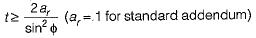∴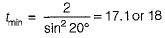QUESTION: 2

### For a single reduction spur gear, the gear ratio is 10 to 1 and the center distance is 330 mm. The nearest standard module if no interference is to occur, will be

Solution: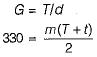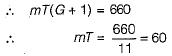Minimum number of teeth to avoid interference, T = 18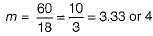QUESTION: 3

### For helical gears, the helix angle generally ranges from

Solution:
QUESTION: 4

For helical gears, the circular pitch Pc, the normal pitch Pn and the helix angle a are related by the identity

Solution: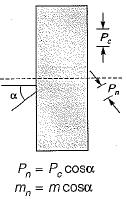QUESTION: 5

Consider the following statements:
1. A stub both has working depth larger than that of a full depth tooth.
2. The path of contact for involute gears is an arc of a circle.
Which of these statements is/are correct?

Solution:

1. A stub tooth has working depth smaller than that of a full depth tooth.
2. The path of contact for involute gears is a straight line.

QUESTION: 6

The ends of gears are made slightly thinner (crowning). This is done

Solution:
QUESTION: 7

The maximum efficiency of worm and worm wheel system is
where φ is the friction angle

Solution:
QUESTION: 8

Tumbler gears are

Solution:
QUESTION: 9

The size of Gear is specified by

Solution:

Size of gear is specified by its pitch and pitch can be classified as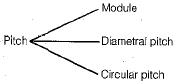Hence circular pitch, diametral pitch and module represents size of gear.

QUESTION: 10

If D is pitch circle diameter and T is the number of teeth then its circular pitch is

Solution: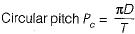where
D = Pitch circle diameter
T = Number of teeth